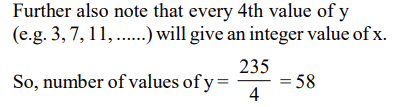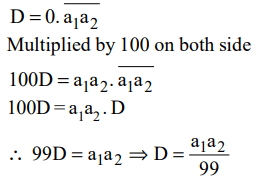## Number System Questions for CAT

1. When $7^{84}$ is divided by 342, what is the remainder?
a) 0
b) 1
c) 49
d) 341

Explanation:2. A, B, C are three distinct digits. AB is a two digit number and CCB is a three digit number such that (AB)2 = CCB where CCB > 320. What is the possible value of the digit B?
a) 1
b) 0
c) 3
d) 9

Explanation: (AB)2 = CCB.
The only number satisfying the given condition
212 = 441.
So, B = 1.

3. For the given pair (x, y) of positive integers, such that 4x – 17y = 1 and x $\leq$ 1000, how many integer values of y satisfy the given conditions
a) 55
b) 56
c) 57
d) 58

Explanation:4. Convert 1982 in base 10 to base 12
a) 1129
b) 1292
c) 1192
d) 1832

Explanation:5. Let D be a recurring decimal of the form $D=0.a_{1}a_{2}a_{1}a_{2}a_{1}a_{2}....$     where $a_{1}$ and $a_{2}$ lie between 0 and 9. Further at most one of them is zero. Which of the following numbers necessarily produces an integer when multiplied by D?
a) 18
b) 198
c) 100
d) 288

Explanation:Required number should be the multiple of 99. So we can get an integer when multiplied by D. Hence, 198 is the required number.

6. P is the product of all the prime numbers between 1 to 100. Then the number of zeroes at the end of P are
a) 1
b) 24
c) 0
d) None of these

Explanation: There are only 2 prime numbers 5 & 2 between 1 & 100 which when multiplied will give zero in the end. Thus there will be only one zero at the end of the product of given number.

7.N = 1421 × 1423 × 1425 what is the remainder when N is divided by 12?
a) 0
b) 1
c) 3
d) 9

Explanation: N = 1421*1423*1425, when these numbers are divided by 12 we have remainders as 5, 7, 9. The product of remainders when divided by 12 gives 3 as its remainder. Thus when N divided by 12 remainder is 3

8. $x_{n}$ is either –1 or 1 & $n\geq 4$  ; If x1x2x3x4+x2x3x4x5+x3x4x5x6+....xnx1x2x3 = 0 then n can be
a) odd
b) even
c) prime
d) can't be determined

Explanation: Every term in the question is either 1 or –1. In order to have zero the number of terms must be even. Note that there are n number of terms. (since the first term in each product varies from x1 to xn ). So n has to be even

9. There are two integers 34041 and 32506, when divided by a three-digit integer n, leave the same remainder. What is the value of n?
a) 298
b) 307
c) 461
d) can’t be determined

Explanation: Let the common remainder be x. Then numbers (34041 – x) and (32506 – x) would be completely divisible by n.
Hence the difference of the numbers (34041 – x) and (32506 – x) will also be divisible by n
or (34041 – x – 32506 + x) = 1535 will also be divisible by n.
Now, using options we find that 1535 is divisible by 307

10. If x, y and z are odd integers then which of the following is necessarily false?
a) xyz is odd
b) (x – y) z is even
c) (x – y) (z + y) x is even
d) (x – y – z) (x + z) is odd